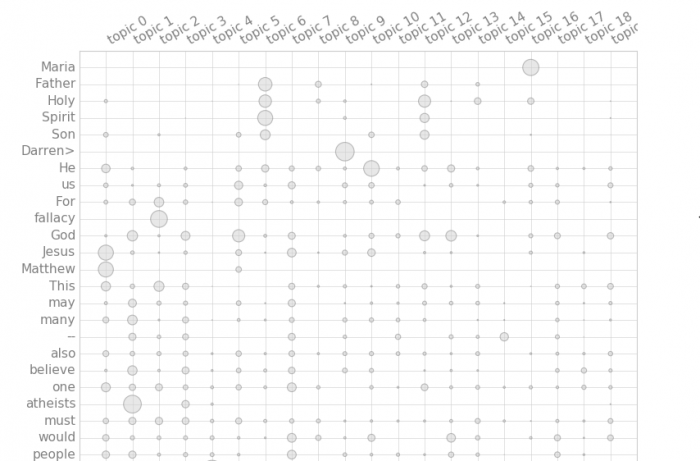# Topic Modeling Python and Textacy Example

Topic modeling is automatic discovering the abstract “topics” that occur in a collection of documents. It can be used for providing more informative view of search results, quick overview for set of documents or some other services.

## Textacy

In this post we will look at topic modeling with textacy. Textacy is a Python library for performing a variety of natural language processing (NLP) tasks, built on the high-performance spacy library.
It can flexibly tokenize and vectorize documents and corpora, then train, interpret, and visualize topic models using LSA, LDA, or NMF methods. 
Textacy is less known than other python libraries such as NLTK, SpaCY, TextBlob  But it looks very promising as it’s built on the top of spaCY.

In this post we will use textacy for the following task. We have group of documents and we want extract topics out of this set of documents. We will use 20 Newsgroups dataset as the source of documents.

## Code Structure

Our code consist of the following steps:
Get data. We will use only 2 groups (alt.atheism’, ‘soc.religion.christian’).
Tokenize and remove some not needed characters or stopwords.
Vectorize.
Extract Topics. Here we do actual topic modeling. We use Non-negative Matrix Factorization method. (NMF)
Output graph of terms – topic matrix.

## Output

Below is the final output plot.Topic modeling with textacy

Looking at output graph we can see term distribution over the topics. We identified more than 2 topics. For example topic 2 is associated with atheism, while topic 1 is associated with God, religion.

While better data preparation is needed to remove few more non meaningful words, the example still showing that to do topic modeling with textacy is much easy than with some other modes (for example gensim). This is because it has ability to do many things that you need do after NLP versus just do NLP and allow user then add additional data views, heatmaps or diagrams.

Here are few links with topic modeling using LDA and gensim (not using textacy). The posts demonstrate that it is required more coding comparing with textacy.
Topic Extraction from Blog Posts with LSI , LDA and Python
Data Visualization – Visualizing an LDA Model using Python

## Source Code

Below is python full source code.

```categories = ['alt.atheism', 'soc.religion.christian']

#Loading the data set - training data.
from sklearn.datasets import fetch_20newsgroups

newsgroups_train = fetch_20newsgroups(subset='train', shuffle=True, categories=categories, remove=('headers', 'footers', 'quotes'))

# You can check the target names (categories) and some data files by following commands.
print (newsgroups_train.target_names) #prints all the categories
print("\n".join(newsgroups_train.data.split("\n")[:3])) #prints first line of the first data file
print (newsgroups_train.target_names)
print (len(newsgroups_train.data))

texts = []

labels=newsgroups_train.target
texts = newsgroups_train.data

from nltk.corpus import stopwords

import textacy
from textacy.vsm import Vectorizer

terms_list=[[tok  for tok in doc.split() if tok not in stopwords.words('english') ] for doc in texts]

count=0
for doc in terms_list:
for word in doc:
print (word)
if word == "|>" or word == "|>" or word == "_" or word == "-" or word == "#":
terms_list[count].remove (word)
if word == "=":
terms_list[count].remove (word)
if word == ":":
terms_list[count].remove (word)
if word == "_/":
terms_list[count].remove (word)
if word == "I" or word == "A":
terms_list[count].remove (word)
if word == "The" or word == "But" or word=="If" or word=="It":
terms_list[count].remove (word)
count=count+1

print ("=====================terms_list===============================")
print (terms_list)

vectorizer = Vectorizer(tf_type='linear', apply_idf=True, idf_type='smooth')
doc_term_matrix = vectorizer.fit_transform(terms_list)

print ("========================doc_term_matrix)=======================")
print (doc_term_matrix)

#initialize and train a topic model:
model = textacy.tm.TopicModel('nmf', n_topics=20)
model.fit(doc_term_matrix)

print ("======================model=================")
print (model)

doc_topic_matrix = model.transform(doc_term_matrix)
for topic_idx, top_terms in model.top_topic_terms(vectorizer.id_to_term, topics=[0,1]):
print('topic', topic_idx, ':', '   '.join(top_terms))

for i, val in enumerate(model.topic_weights(doc_topic_matrix)):
print(i, val)

print   ("doc_term_matrix")
print   (doc_term_matrix)
print ("vectorizer.id_to_term")
print (vectorizer.id_to_term)

model.termite_plot(doc_term_matrix, vectorizer.id_to_term, topics=-1,  n_terms=25, sort_terms_by='seriation')
model.save('nmf-10topics.pkl')

```